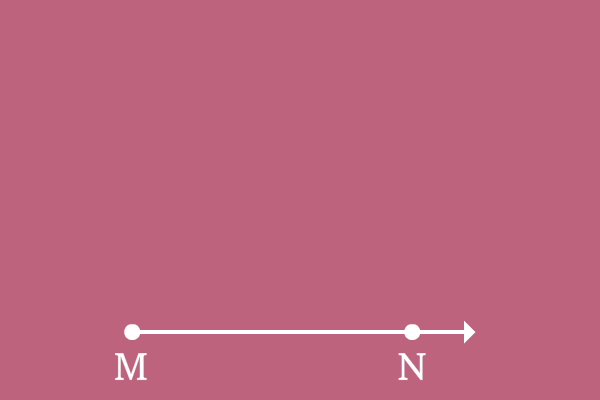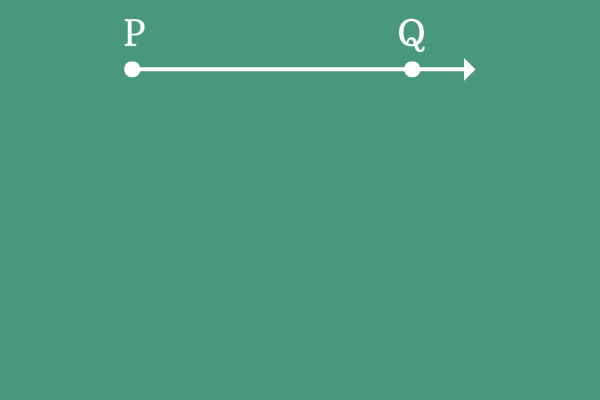# Sense of an angle

## Definition

The direction of rotation of a ray from its initial position to final position is called sense of angle.

An angle is usually formed by a ray by rotation but the rotation of a ray is possible geometrically either clockwise or anticlockwise to form an angle geometrically. To understand how it is formed geometrically, the direction of rotation of a ray to form an angle is denoted by either plus sign or minus sign.

The plus sign or minus sign are actually written before the angle.

1. A plus sign $(+)$ or without a plus sign before the angle represents that the angle is made by a ray in anticlockwise direction.
2. A minus sign $(-)$ before the angle represents that the angle is made by a ray in clockwise direction.

The symbolic representation senses how the angle is formed geometrically.

### Classification

According to concept of sense of angle, the angles are classified into two types.

1

#### Positive anglePositive angle is an angle and it is formed when a ray is rotated in clockwise direction from its initial position to final position.

2

#### Negative angleNegative angle is an angle and it is formed when a ray is rotated in anticlockwise direction from its initial position to final position.

Latest Math Topics
Jun 26, 2023
Jun 23, 2023

Latest Math Problems
Jul 01, 2023
Jun 25, 2023
###### Math Questions

The math problems with solutions to learn how to solve a problem.

Learn solutions

Practice now

###### Math Videos

The math videos tutorials with visual graphics to learn every concept.

Watch now

###### Subscribe us

Get the latest math updates from the Math Doubts by subscribing us.